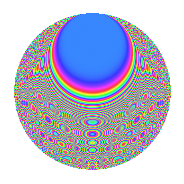# Properties

 Label 504.2.caLevel 504 Weight 2 Character orbit ca Rep. character $$\chi_{504}(5,\cdot)$$ Character field $$\Q(\zeta_{6})$$ Dimension 184 Newform subspaces 1 Sturm bound 192 Trace bound 0

# Related objects

## Defining parameters

 Level: $$N$$ = $$504 = 2^{3} \cdot 3^{2} \cdot 7$$ Weight: $$k$$ = $$2$$ Character orbit: $$[\chi]$$ = 504.ca (of order $$6$$ and degree $$2$$) Character conductor: $$\operatorname{cond}(\chi)$$ = $$504$$ Character field: $$\Q(\zeta_{6})$$ Newform subspaces: $$1$$ Sturm bound: $$192$$ Trace bound: $$0$$

## Dimensions

The following table gives the dimensions of various subspaces of $$M_{2}(504, [\chi])$$.

Total New Old
Modular forms 200 200 0
Cusp forms 184 184 0
Eisenstein series 16 16 0

## Trace form

 $$184q - 2q^{4} - 6q^{6} - 2q^{7} - 2q^{9} + O(q^{10})$$ $$184q - 2q^{4} - 6q^{6} - 2q^{7} - 2q^{9} - 6q^{10} + 12q^{12} - 18q^{14} - 2q^{15} - 2q^{16} + 12q^{18} - 6q^{22} - 6q^{23} - 12q^{24} + 78q^{25} - 6q^{26} - 8q^{28} + 7q^{30} - 6q^{33} + 6q^{34} + 22q^{36} - 33q^{38} - 8q^{39} - 18q^{40} - 42q^{42} - 9q^{44} + 2q^{46} - 12q^{47} + 9q^{48} - 2q^{49} + 9q^{50} + 21q^{52} + 33q^{54} + 18q^{56} + 4q^{57} - 3q^{58} - 59q^{60} + 12q^{62} - 72q^{63} - 8q^{64} - 42q^{66} - 18q^{68} - 27q^{70} + 12q^{72} - 12q^{73} - 57q^{74} + 12q^{76} + 19q^{78} - 4q^{79} - 57q^{80} - 18q^{81} + 69q^{84} - 27q^{86} - 6q^{87} + 9q^{88} - 24q^{89} - 75q^{90} - 36q^{92} - 45q^{96} - 45q^{98} + O(q^{100})$$

## Decomposition of $$S_{2}^{\mathrm{new}}(504, [\chi])$$ into newform subspaces

Label Dim. $$A$$ Field CM Traces $q$-expansion
$$a_2$$ $$a_3$$ $$a_5$$ $$a_7$$
504.2.ca.a $$184$$ $$4.024$$ None $$0$$ $$0$$ $$0$$ $$-2$$

## Hecke Characteristic Polynomials

There are no characteristic polynomials of Hecke operators in the database